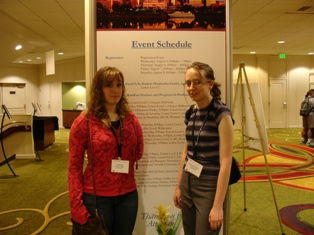Are you interested in doing undergraduate research in mathematics, attend a conference, and publish your journal paper? If so, follow the links to the below for information about undergraduate research opportunities, conferences, and journals.

## Journals of Undergraduate Research in Mathematics

Below is a list of Mathematics faculty members at UMW involved with undergraduate research, with topics of interest.

Yuan-Jen Chiang:

1. The applications of fractional calculus in mathematics and physics
2. Minimal surfaces
3. The applications of PDE/Fourier Analysis
4. Differential geometry and its applications to physics
5. Elliptic partial differential equations and harmonic maps
6. Harmonic maps and applications to physics
7. Einstein’s Equations and Their Applications
8. Constant Mean Curvature Surfaces and Their Applications
9. Gauss-Cadazzi- Mainardi Equations and Their Applications
10. Gauss-Bonnet Formulas and Their Applications

Randall Helmstutler:

My undergraduate research interests lie primarily in generalizations of parts of abstract algebra and topology.  My true research interests are in an area called category theory, which is an attempt to give axiomatic approaches that unify algebra, topology, and other parts of purely theoretical mathematics.  I have had several students complete projects in category theory and would be interested in doing more undergraduate research in this area.  To see examples of my former students’ work, visit my student research page.

Debra L. Hydorn:

I am interested in working with students on projects in multivariate statistics (e.g., regression analysis, analysis of variance, variable reduction techniques).  Most projects will include the derivation of some theoretical results and simulations to verify distributional properties.  I am also interested in working with students in the use of spatial statistics, particularly in environmental applications.  Some course work in statistics is needed, preferably MATH 381, and some background in programming is helpful but not required.  To learn more about the kinds of projects I have mentored please visit my undergraduate research page.

Jangwoon “Leo” Lee:

I have various undergraduate research problems in applied mathematics. In a project, for instance, you would consider a mathematical model equation (e.g., differential equations or (stochastic) partial differential equations) of physical phenomenon. Then you would try to solve it by some mathematical methods (if possible) and by numerical methods using programming languages such as MATLAB (including built-in solvers in MATLAB) to make predictions about how the physical phenomenon will behave in different circumstances and/or evaluate the performance of numerical solvers of the model equation. In this project, you would learn how to analyze a real-life problem using the mathematical language that is used by many people in industry and government. This would be a great experience and also would give a great opportunity to you who may be interested in seeking employment at places like Dahlgren. My former students’ projects can be found in here.

Suzanne Sumner:

I have two projects in mind for undergraduate research.

1. Competing Species Models

Competing species forestry models use differential equations to examine the long-term population levels with two types of species of trees. These species are either pioneer (those trees that are deprivation intolerant) and climax (those trees that receive a benefit from having other trees nearby.) In particular, the primary concern is when the long-term tree population values level off or fluctuate. Hopf bifurcations mark a change in the dynamics from stable to unstable scenarios.

2. Honey Bee Biology Models

In recent years honey bees have been parasitized by two species of mites, the tracheal mite and the varroa mite. These mites have decimated numerous colonies, severely impacting the areas of agriculture that rely on honey bee pollination. Difference equation models treat the parasitism as a Susceptible-Infected-Removed (SIR) model as in earlier work by Dr. Wyatt A. Mangum. Some bees carry desirable traits that allow them to withstand mite infestation. Here the primary concern is determining the proportion of bees with these traits that a colony must have so that the overall percent infestation decreases. Bifurcation surfaces separate unstable scenarios where percent mite infestation increases from stable situations where percent infestation decreases.